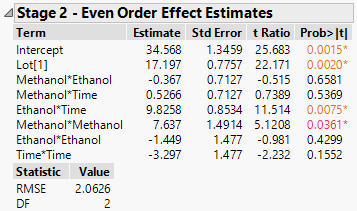Publication date: 11/29/2021

## Stage 2 - Even Order Effect Estimates

The Even Order Effect Estimates report lists second-order effects that are identified as active. Active second-order effects are identified using the guided variable selection procedure or forward selection as described in Stage 2 Methodology. The block effect (if one is included) is also listed, whether it is significant or not.

Figure 8.9 Stage 2 ReportTerm

The block factor and second-order effects identified as active.

Estimate

Parameter estimates for a regression fit of Y on the Stage 2 second-order effects defined by Y2nd. See Decomposition of Response.

Std Error

The standard error of the estimate, computed using the Stage 2 RMSE.

t Ratio

The Estimate divided by its Std Error.

Prob>|t|

The p-value computed using the t Ratio and the degrees of freedom for error (DF).

RMSE

The square root of the mean square error that results from the Stage 2 analysis. RMSE is estimated as the residual variance from Y2nd after fitting the second order effects identified as active. See Decomposition of Response.

DF

The degrees of freedom associated with the error estimate used to construct RMSE.